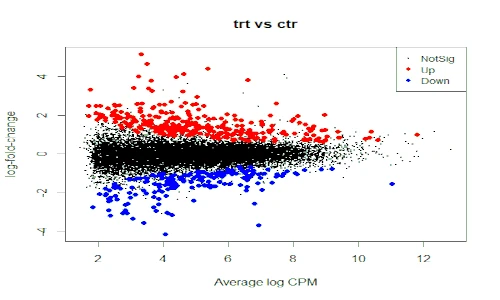# Make discoveries with your data

## Subscribe to get new article to your email when published

* indicates required

### Recent posts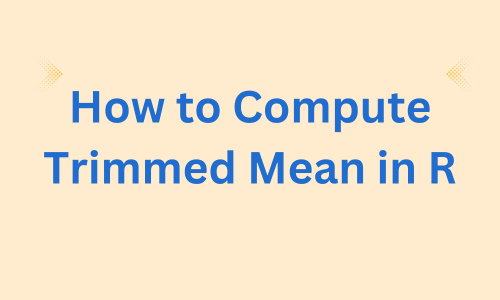## How to Compute Trimmed Mean in R?

Learn how to calculate trimmed mean in R with several examples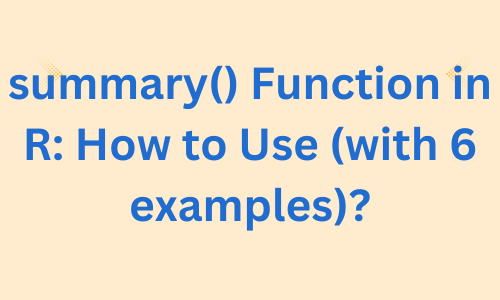## `summary()` Function in R: How to Use (With 6 Examples)?

How to use summary() function in R to get summarise the fitted model results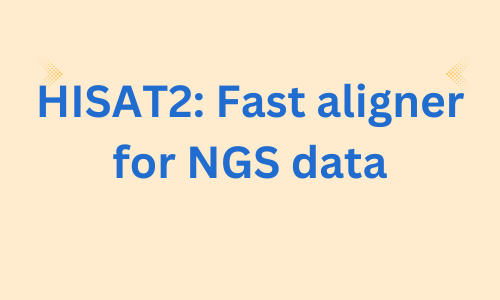## HISAT2: Fast Aligner for NGS Data (complete Tutorial)

This tutorial explains how to use HISAT2 for aligning NGS reads to genome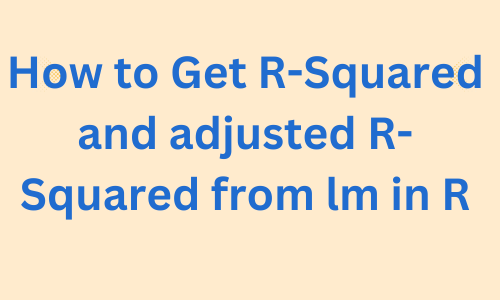## How to Get R-Squared and Adjusted R-Squared From `lm` in R

Extract R-Squared and adjusted R-Squared from lm summary statistics after fitting regression model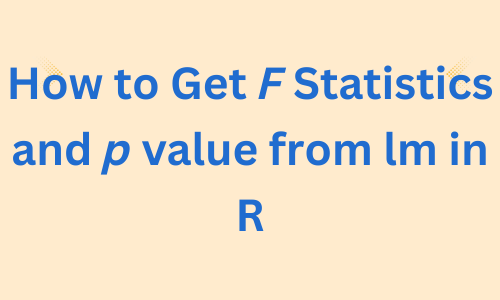## How to Get F Statistics and p Value From `lm` in R

Extract F Statistics and p value from lm summary statistics after fitting regression model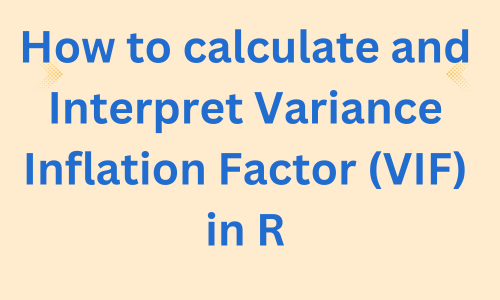## How to Calculate VIF in R

This article describes how to calculate the Variance Inflation Factor (VIF) to detect multicollinearity in R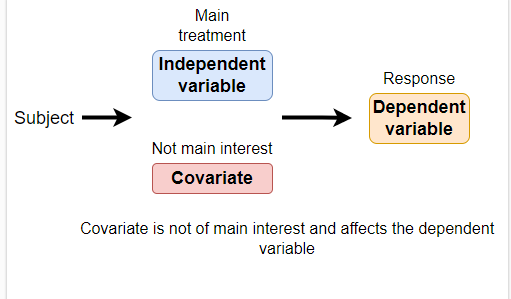## Covariate in Statistics: Definition and Examples

Explanation of covariate in statistics, covariate examples, and how to use covariate in statistical analysis## Two-pass alignment of RNA-seq reads with STAR

Complete tutorial on how to use STAR aligner in two-pass mode for mapping RNA-seq reads to genome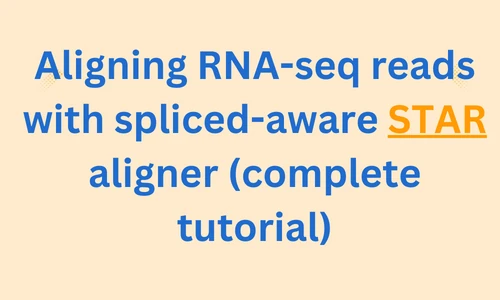## Mastering RNA-seq Read Alignment With STAR

Learn how to align RNA-seq reads with STAR for accurate gene expression analysis in bioinformatics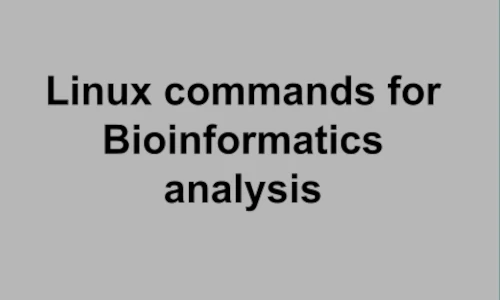## Linux for Bioinformatics (for beginners)

Learn Linux command lines for Bioinformatics analysis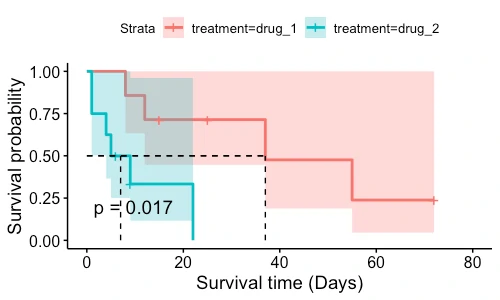## Survival analysis (Kaplan–Meier, Cox proportional hazards, and Log-rank test methods)